Example Questions

← Previous 1 3 4 5 6 7 8 9 16 17

Example Question #1 : Percentage

We asked 1000 people their preferences of movie genres. 7.5% of those asked preferred horror movies, 35% preferred romantic films, 20% preferred dramas and 37.5% preferred comedies. How many people liked horror films?

7.5

750

37.5

75

35

75

Explanation:

People who prefer horror films = 1000 people x 7.5% = 1000 x 0.075 = 75 people

Example Question #1 : How To Find A Fraction From A Percentage

There are 20 students in a class. 35 percent of the students in the class are boys. What fraction of the class is girls?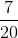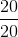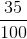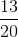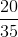Explanation:

There are 20 total students in the class. 35% of 20 is 7. There are 7 boys in the class.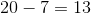girls in the class.

Example Question #1 : Percentage

Which of the following represents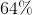as a fraction?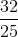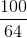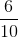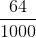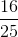Explanation:

To represent a percentage as a fraction, keep the percentage in the numerator and putin the denominator.

Therefore: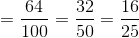Example Question #3 : Fractions And Percentage

Which of the following fractions is equivalent to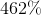Explanation:

To convert an integer percentage into an improper fraction, set the percentage as a fraction overand simplify as far as you can. At that point, simply convert the improper fraction into a mixed fraction.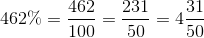Example Question #1 : Percentage

A politician promises to spend up to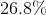of his budget on improving roads. Which of the following is closest to the fraction of their budget the politician must devote to road improvement?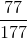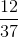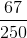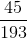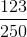Explanation:

To convert a decimal percentage into a fraction, first convert it to a decimal: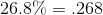Now, set ths as a fraction with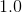as the denominator (expanding to as many significant digits as you need to line the fraction up):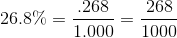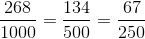Example Question #5 : Fractions And Percentage

Convert to a fraction: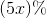Explanation:

To convert a percentage to a fraction, divide the percentage by 100, then simplify. The presence of a variable does not significantly affect this process.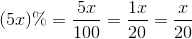Example Question #1 : How To Find Percentage From A Fraction

Luke went to the grocery store. It took him 15 minutes to drive to the store from his house, 30 minutes to shop, 5 minutes to put groceries in his car, and 20 minutes to drive home due to traffic.

What percentage of the trip did Luke spend inside his car?

75%

35%

45%

15%

50%

50%

Explanation:

You take 15 + 20 = 35 minutes as total time spent in the car.

Then, you take 15 + 20 + 5 + 30 = 70 minutes as total trip time.

We take (35/70) x 100% = 50%

Example Question #1 : Percentage

There are 300 sandwiches at a company-wide picnic. If half of the sandwiches are tuna, and the provider mistakenly uses expired tuna in three-fifths of those sandwiches, what percentage of total sandwiches still remain edible?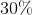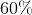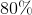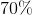Explanation:

This multi-part question makes use of both fractions and percentages, as well as some tricky language if you are not paying close attention.

First off, determine what three-fifths of the 150 tuna sandwiches is: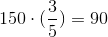Now, refer back to the question, which asks how many TOTAL sandwiches (tuna and non-tuna) still remain edible. This is simple arithmetic.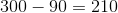Finally, find what percentage this equals: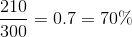Example Question #1 : Percentage

Express the fraction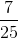as a percentage.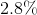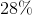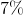Explanation:

Converting a fraction to a percentage can be done in two ways.  The first way involves recalling that a percent is just a fraction of 100 (per cent).  Therefore, the percent equivalent tois the numerator of the equivalent fraction whose denominator is 100.  In order to convertto a fraction over 100, I need to multiply the numerator and denominator each by 4.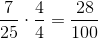The other approach is to convert the fraction to a decimal first by dividing 7 by 25.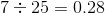To convert a decimal to a percent, just shift the decimal point two places to the right.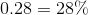With this method, our answer is again 28%.

Example Question #1 : Percentage

Convert to a percentage: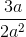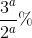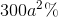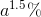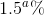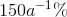Explanation:

Despite the presence of a variable, the rule remains the same: To convert a fraction to a percentage, multiply the numerator by 100 and solve the fraction.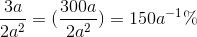← Previous 1 3 4 5 6 7 8 9 16 17

All ACT Math Resources